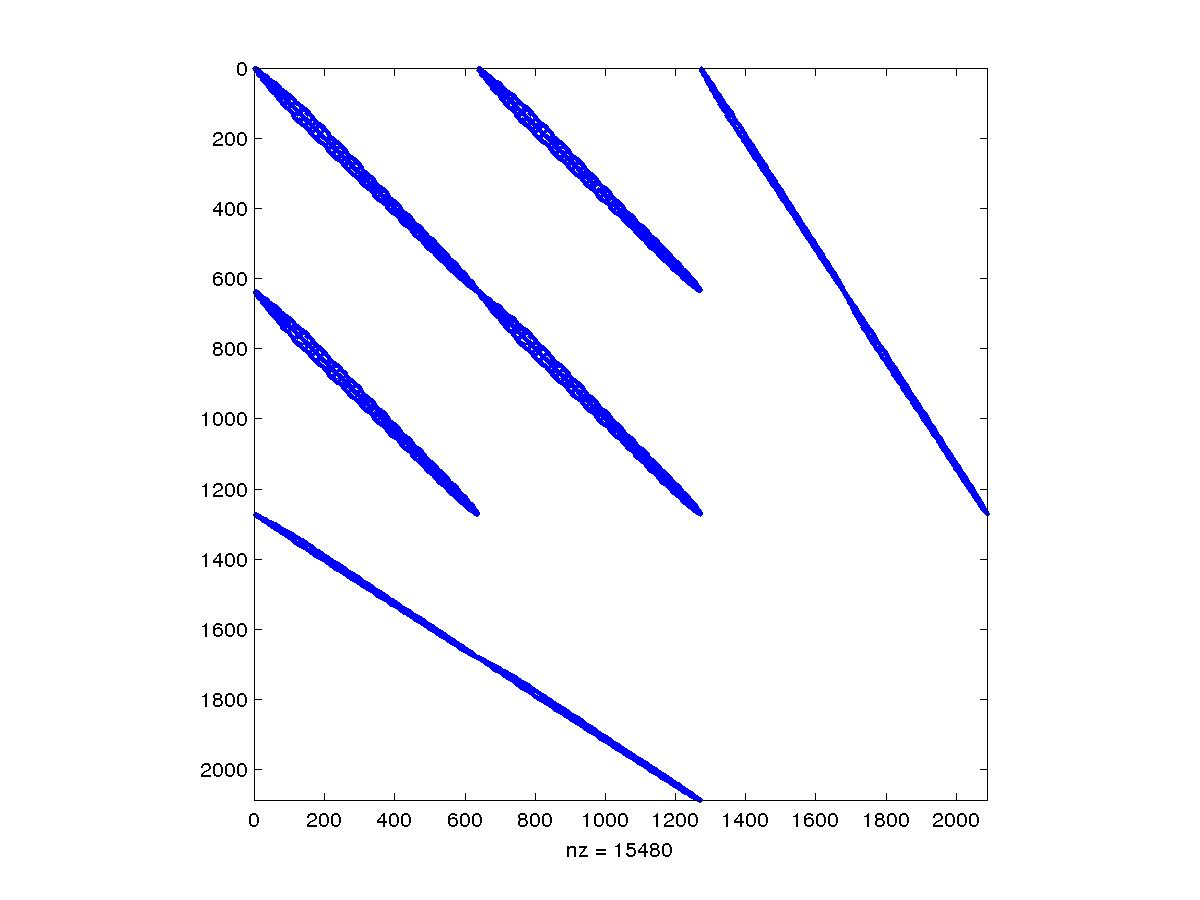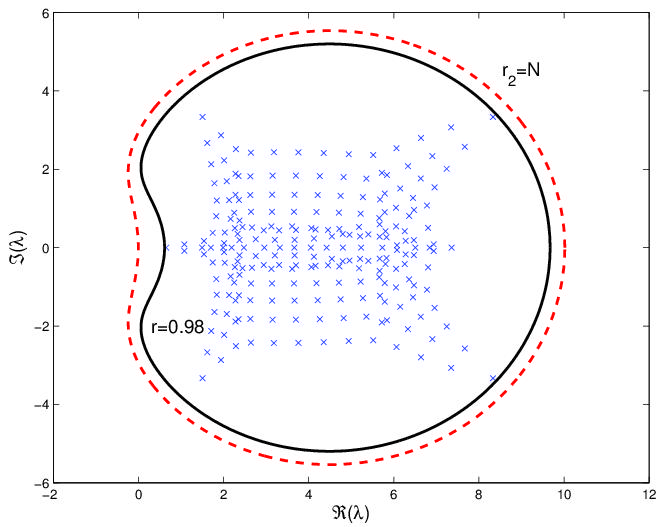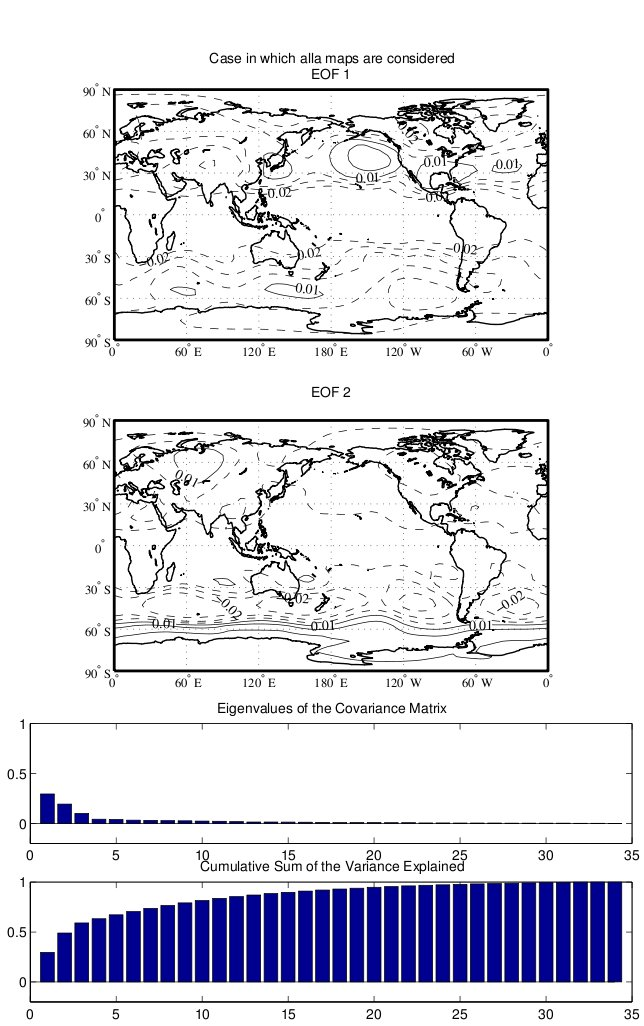# Valeria Simoncini. Research Interests and Problem Solving

## Problem Solving:

### Development and analysis of efficient solution strategies for large algebraic linear systems: symmetric and nonsymmetric systems stemming from FD, FE, etc methods for elliptic PDEsSaddle point problems from (PDE-)Constrained Optimization, Mixed formulations, etc. (for the plot see )Relaxed approximation of matrix computations for efficiency (for the plot see ); Matrix analysis and solution strategies for PDE discretization methodsSparsity pattern analysis and its use in adaptive discretization methodologies (for the plot see );Definition of key matrices in mimetic FD methods for polygonal/polyhedral meshes (see );Numerical solution of ill-posed elliptic problems by means of matrix functions (for the plot see ); Numerical treatment of multiparameter and stochastic PDEs for uncertainty quantificationParameter-dependent problems and Model Order Reduction (for the plot see ,);Parameterized linear equations and stochasticity parameters in Structure modelling (for the plots see )PDEs with random inputs (for info see , data from SIFISS); Numerical modeling of life science phenomenaStructured time-dependent systems in Electrocardiology Modelling (for the plot see )Spectral sensitivity in dynamical systems modelling Gene Networks (for the plot see ) Matrix Analysis and algorithms in Dynamical systems and ControlNumerics associated with Lyapunov, Sylvester and Riccati algebraic equations (for the plot see ); Matrix Computations in StatisticsPrincipal Component Analysis in Athmospheric studies (for the plot see )Covariance matrix approximation (for the plot see );

Brief selection of References (a complete list here ):

A. Navarra and V. Simoncini,A Guide to Empirical Orthogonal Functions for Climate Data Analysis 2010, 200 p. With online files/update., Hardcover, Springer.

M. Benzi and V. Simoncini, Decay bounds for functions of matrices with banded or Kronecker structure SIAM J. Matrix Analysis and Appl. 2015.

I. Perugia and V. Simoncini, Block-diagonal and indefinite symmetric preconditioners for mixed finite element formulations Numerical Linear Algebra with Appl. v. 7, n.7--8 (2000), pp. 585--616.

V. Simoncini, The Extended Krylov subspace for parameter dependent systems Applied Num. Math. v.60 n.5 (2010) 550-560.

Gianluca Barbella, Federico Perotti and V. Simoncini Block Krylov subspace methods for the computation of structural response to turbulent wind Comput. Meth. Applied Mech. Eng. (CMAME), (2011), v. 200(23-24), pp. 2067-2082. MAME

Catherine E. Powell , David Silvester , and V. Simoncini An efficient reduced basis solver for stochastic Galerkin matrix equations MIMS EPrint 2015.64, University of Manchester, UK.

V.Druskin and V. Simoncini, Adaptive rational Krylov subspaces for large-scale dynamical systems Systems & Control Letters, 60 (2011), pp. 546-560.

V. Simoncini and F. Perotti, On the numerical solution of (l2 A + l B + C) x = b and application to structural dynamics SIAM J. Sci. Comput. v. 23 n.6 (2002) pp. 1876-1898.

M. Pennacchio V. Simoncini, Fast structured AMG Preconditioning for the bidomain model in electrocardiology SIAM J. Scient. Computing., v. 33, n.2, pp. 721-745 (2011).

Liliana Ironi, Luigi Panzeri , Erik Plahte and V. Simoncini Dynamics of actively regulated gene networks Physica D, 240 (2011), pp.779-794.

Claudio Canuto , V. Simoncini and Marco Verani On the decay of the inverse of matrices that are sum of Kronecker products Linear Algebra and its Applications, Volume 452, 1 July 2014, Pages 21-39.

V. Simoncini and Daniel B. Szyld , Theory of Inexact Krylov Subspace Methods and Applications to Scientific Computing SIAM J. Scient. Computing, v.25, n.2 (2003), pp. 454-477.

Franco Brezzi , Konstantin Lipnikov and V. Simoncini, A family of mimetic finite difference methods on polygonal and polyhedral meshes M3AS: Mathematical Models and Methods in Applied Sciences, v.15 n.10 (2005) pp. 1533-1552.

V. Simoncini and Vladimir Druskin, Convergence analysis of projection methods for the numerical solution of large Lyapunov equations SIAM J. Numerical Analysis. Volume 47, Issue 2,pp. 828-843 (2009).

Lars Elde'n and V. Simoncini, Solving Ill-Posed Cauchy Problems by a Krylov Subspace Method Inverse Problems, v.25, n.6 (June 2009).